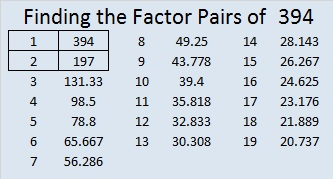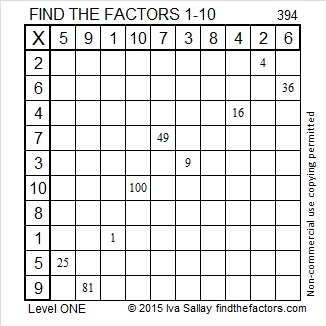# Pythagorean Triples with 394

Since 394 is an even number not divisible by 4, it can’t be part of a primitive Pythagorean triple. Scroll down past the puzzle to see its factors and its two non-primitive triples.Print the puzzles or type the factors on this excel file: 10 Factors 2015-02-16

• 394 is a composite number.
• Prime factorization: 394 = 2 x 197
• The exponents in the prime factorization are 1 and 1. Adding one to each and multiplying we get (1 + 1)(1 + 1) = 2 x 2 = 4. Therefore 394 has exactly 4 factors.
• Factors of 394: 1, 2, 197, 394
• Factor pairs: 394 = 1 x 394 or 2 x 197
• 394 has no square factors that allow its square root to be simplified. √394 ≈ 19.849To find Pythagorean triples with the number 394, first divide it in half to get 197. Since 197 is 14² + 1, we know it is the hypotenuse of the triple formed from 2(14 x 1), 14² – 1, and 14² + 1 or (28-195-197). If we multiply that triple by 2, we get (56-390-394).

Prime number 197 is also the short leg of the primitive Pythagorean triple, (197-19404-19405). Doubling each element of that triple gives us (394-38808-38810).This site uses Akismet to reduce spam. Learn how your comment data is processed.